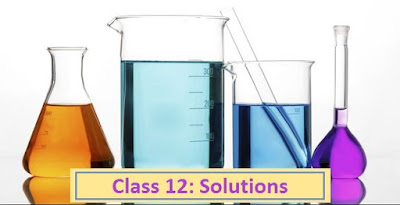# Important Questions for Class 12 Chemistry, Chapter-‘SOLUTION’

May 10, 2020

Last Updated on September 4, 2021 by Science Master

## SOLUTION

### Class-12## Important Questions for Class 12 Chemistry, Chapter-‘SOLUTION’

A homogeneous mixture of two or more solid, liquid or gaseous substances, the amount of each of which can be changed up to a certain limit, is called a solution.
The solution chapter is an important chapter in twelfth grade chemistry. Below are some important questions from this solution chapter which will be useful for students to prepare for various exams.

1. Write Henry’s formula. This formula does not apply to any type of gas.

2. What is liquid vapor pressure?

3. Why does the vapor pressure of the liquid increase when the temperature rises?

4. Write Roulette’s formulas for the vapor pressure of a solution.

5. What is the ideal solution?

6. What is a non-ideal solution?

7. What is the relative degradation of vapor pressure?

8. Write Raoult’s formulas for the relative degradation of vapor pressure.

9. From Raoult’s formula for the relative degradation of vapor pressure, show that the vapor pressure of a solution varies proportionally with the mole fraction of the pure solvent.

10. What is aziotropic mixture called?

11. What is numerical religion or concurrent or collegiate religion?

12. According to Raoult’s formula for the vapor pressure of a solution, show that the relative degradation of vapor pressure is equal to the mole fraction of the solution.

13. Why does the relative degradation of vapor pressure not depend on temperature?

14.. Why is the relative decline of vapor pressure a numerical religion?

15. How to determine the molecular importance of a solution with Raoult’s formula for the relative degradation of vapor pressure.

16. Write Raoult’s formulas for the development of the boiling point of a solution.

17. Establish the equation for determining the molecular weight of a solution using Raoult’s formula for the development of the boiling point of a solution.

17. Write the applicability of Raoult’s formula regarding the development of crystals.

18. Why is the development of boiling point of light solution a numerical religion?

19. Define molal development constant (kb) of boiling point.

20. Why does the value of kb depend on the nature of the solvent but not on the nature of the solvent?

22. What is Molal freezing degradation constant (kf) called?

23. Why is the freezing point of solution less than that of pure solvent?

24. What is osmotic pressure? What is a semi-permeable screen?

25. Write Vant-haff formulas for osmotic pressure.

26. Establish a mathematical relationship between the osmotic pressure of Vant-haff.

27. Establish the equation for determining the molecular importance of a solution using the Vant-haff formula.

28. What is an isotonic solution?

29. Show that the molar concentration of two isotonic solutions is equal.

30. What is the vant-haff multiplier? What are hypotonic and hypertonic solutions?

31. Write the relation of addition (x) and subtraction dimension (α) of electrolytic solvent with vant-haff factor.

32. Understand what is meant by reverse osmosis pressure.

33. Why is the osmotic pressure of 2% NaCl solution four times greater than that of 1% C6H12O6 solution?

34. Molarity does not depend on temperature but why molarity depends on temperature?

35. Write the equations of different numerical religions for addition and subtraction of solids.

36. Why 0.84% ​​solution of NaCl is used as normal saline?

37. Why do doctors advise patients with high blood pressure to eat less salt?

38. Why should the concentration of the solution that is injected into the vein during injection be equal to the concentration of blood?

39. Why does marine fish die if they are transferred to ponds or rivers?

40. The freezing point of the solvent is proportional to the concentration of the solute dissolved in the solution.

##### 0 thoughts on “Important Questions for Class 12 Chemistry, Chapter-‘SOLUTION’”
1.Subrata Kumar Ghosh says:

Thik e achha